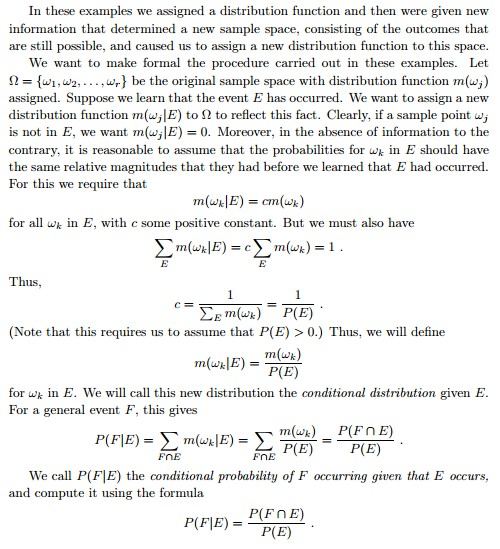Let’s assume there some people in a group.

20% are from France=

30% are from UK

50% of french are boys=

30% of English are boys

Obviously If we choose a person randomly the probability of choosing a french person is 20%.

If I tell you that the chosen person is a boy, What would be the probability of the chosen to be french.

Posterior probability is a revised probability that takes into account new available information.

From the symmetry we know

Posterior Probability

We can also interpret that as

So we can consider as the correction for prior probability   which is called “Prior probability”.

as the result of the other information we have about a condition which is known to be the case.

In the example above we are interested in

If we knew the total proportion of boys the answer would be easy but we don’t.

However,

therefore even without knowing the Probability of B, if we know about B’s posterior probabilities, we can answer the question.

=================================================================================

http://en.wikipedia.org/wiki/Posterior_probability

http://en.wikipedia.org/wiki/Bayes’_theorem

Here is a nice proof for the fundamental conditional probability formula from:

http://www.dartmouth.edu/~chance/teaching_aids/books_articles/probability_book/Chapter4.pdfHypothesis testing for Bayes:

–We have a hypotheses H0 (null),
-We have Alternative Hypothesis H1
–We have data (Y)
–We want to check if the model that we have (H1) fits our data and doesn’t fit H0 (accept H1 / reject H0) or
our data fits (H0)  (Fail to reject H0)

Inferential statistics:

what is the probability that we can reject H0 and accept H1 at some level of significance (alpha, P)

Bayes:

We get some evidence for the model (“likelihood”) and then can even compare “likelihoods” of different models

We want to know the probability of Hypothesis to be true if Observation is observed.

But what is the probability of H0 and what is the probability H1

So the classical P-Value can be interpreted as probability of H0 if O.

But

If H0 is 80% probable (or anything less than 1), while Observation has close to 0.05 chance of happening if H0

This means that if H0 is more likely then a more significant observation is necessary.

This means that if H0 is highly probable then we need a very significant observation to refute it.

If H0 is .85 probable in my mind and an observation is only 1% probable based on it, then the probability of its truth is about 5%.